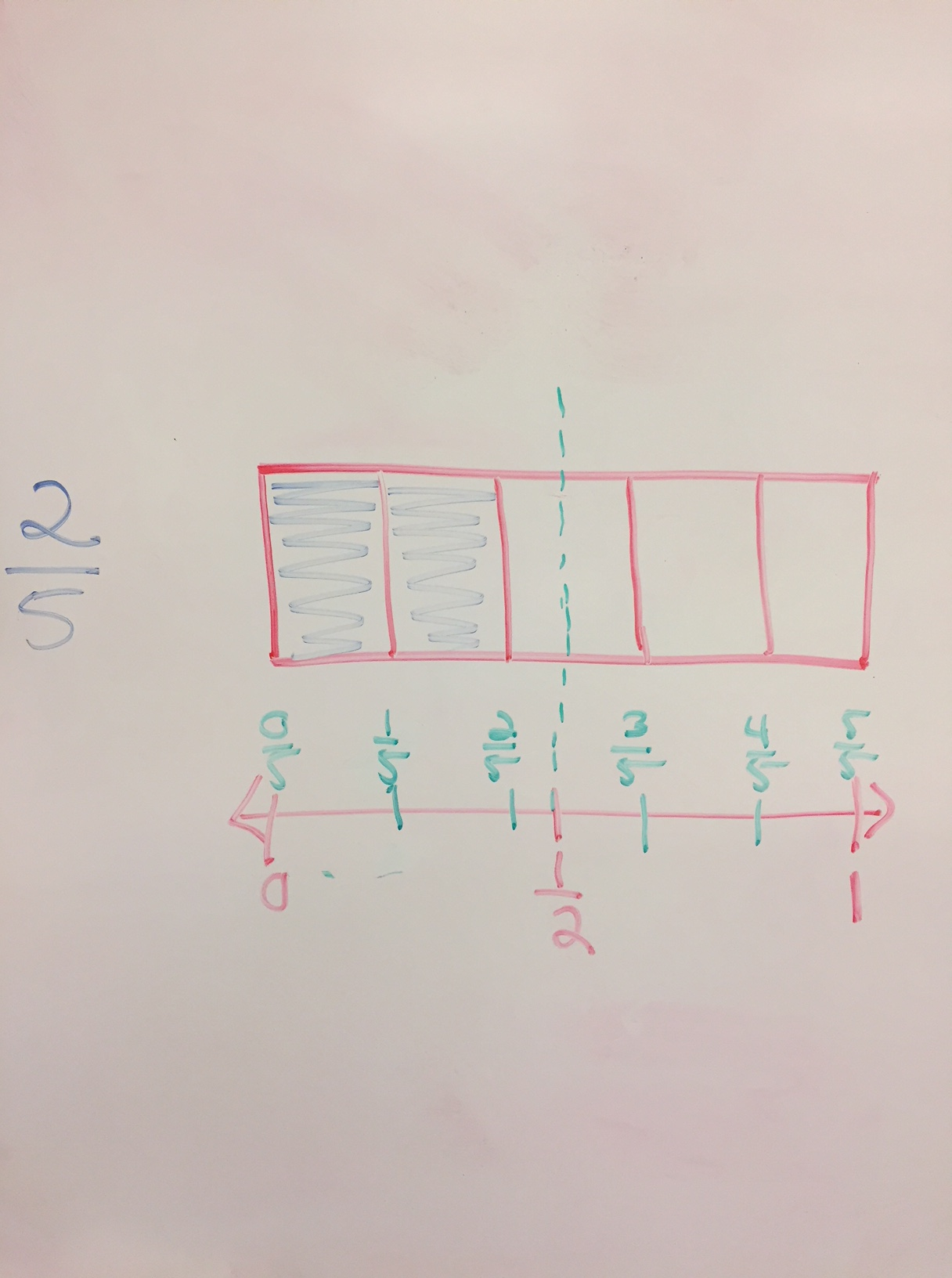# Fourth Grade Math: Using Benchmark Fractions

In addition to our sub sandwich investigation, fourth graders are continuing to explore fraction relationships.  One thing we have been discussing is using benchmarks such as 0, ½, and 1 to think about sizes of fractions.

We have explored the benchmark of a half a great deal.  We looked at different fractions to decide if they were greater than , equal to, or less than a half.  This requires having an understanding of different ways of representing ½.   Students are quite comfortable creating fractions equivalent to a half, such as 5/10, 3/6, and 4/8.

We then pushed this idea further by thinking about whether fractions are closer to 0, ½, or 1.  We used number lines and area models to explore this.  For example, if we have the fraction ⅝, we can see on a number line that it is only ⅛ away from ½ (which can also be written as 4/8), but it is ⅜ away from 1 (which can also be written as 8/8).  When the denominators were even, students had no difficulty with this task. However, then we moved to fractions such as ⅖.  Here, it was a lot more difficult to think about the ½.  Once we lined up our area models with our number lines, it became much clearer.  Students even began to feel comfortable talking about a ½ of a fifth, which we wrote as 0.5/5 or 1/2/5.  They were shocked to learn that we could write a fraction within a fraction! We realized from the models that 2 ½ fifths would be equal to a half (see the picture below), so ⅖ would be closer to a half than 0 since it is only half a fifth away from half and 2 fifths away from zero.